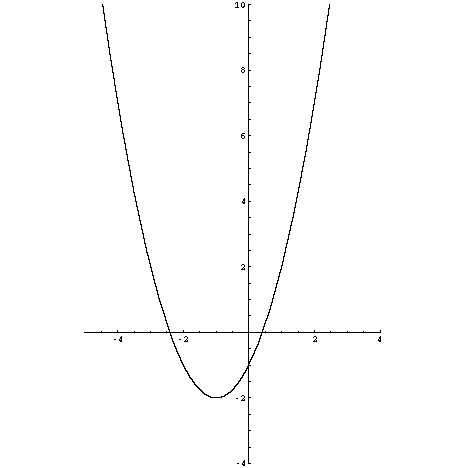A quadratic equation is a polynomial equation whose degree is two. Quadratic equations become a major topic in Algebra.A quadratic function graphed on a coordinate plane.

Although quadratic equations can take many forms, there is a general form that is used most commonly.

(1)
\begin{equation} ax^2+bx+c=0 \end{equation}

In this form, x is the variable of the polynomial. The a, b, and c are the coefficients of the x variable where a is the quadratic term being of the second power, b is the linear term, and the c is the constant term. Of these terms, a cannot be equal to zero or else the equation no longer can be considered quadratic.